# Find Derivatives of Functions in Calculus

Find the derivatives of various functions using different methods and rules in calculus. Several Examples with detailed solutions are presented. More exercises with answers are at the end of this page.

Example 1: Find the derivative of function f given bySolution to Example 1:
Function f is the product of two functions: U = x 2 - 5 and V = x 3 - 2 x + 3; hence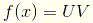We use the product rule to differentiate f as follows: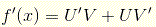where U ' and V ' are the derivatives of U and V respectively and are given by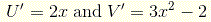Substitute to obtain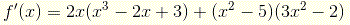Expand, group and simplify to obtain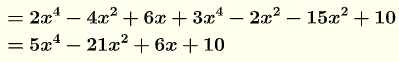Example 2: Calculate the first derivative of function f given by

f(x) = (\sqrt x + 2x)(4x^2-1)

Solution to Example 2:
This function may be considered as the product of function U = √x + 2x and V = 4x2 - 1, hence the use of the product rule
f'(x) = U' V + U V' \\ = (\dfrac{1}{2\sqrt x} + 2)(4x^2-1) + (\sqrt x + 2 x)(8x)
To add the above you need to write all terms as fractions with a common denominator.
f'(x) = \dfrac{(1+2\cdot2\sqrt x)(4x^2-1)+2\sqrt x(8x)(\sqrt x + 2x)}{2\sqrt x}
Expand
f'(x) = \dfrac{4x^2-1+16x^{5/2}-4\sqrt x+16x^2+32x^{5/2}}{2\sqrt x}
and group to obtain the final result for the derivative of f as follows.
f'(x) = \dfrac{48x^{5/2}+20x^2-4x^{1/2}-1}{2\sqrt x}

Example 3: Calculate the first derivative of function f given bySolution to Example 3:
The given function may be considered as the ratio of two functions: U = x2 + 1 and V = 5x - 3 and use the quotient rule to differentiate f is used as followsExpand and group to obtain f'(x) as follows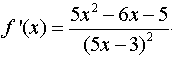Example 4: Calculate the first derivative of function f given bySolution to Example 4:
Function f is the quotient of two functions hence the use of the quotient ruleWrite all terms in the numerator so that they have the same denominator 2 sqrt x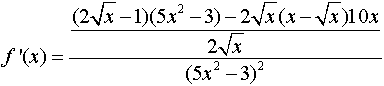Expand and group like terms to obtain f'(x)Example 5: Calculate the first derivative of function f given bySolution to Example 5:
Function f given above may be considered as the product of functions U = 1/x - 3 and V = (x2 + 3)/(2x - 1), and function V may be considered as the quotient of two functions x2 + 3 and 2x - 1. We use the product rule for f and the quotient rule for V as followsSet all terms to a common denominator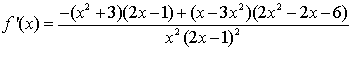Expand and group to obtain the derivative f'.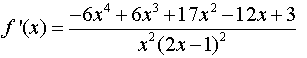Example 6: Calculate the first derivative of function f given bySolution to Example 6:
There are several ways to find the derivative of function f given above. One of them is to consider function f as the product of function U = sqrt x and V = (2x - 1)(x3 - x) and also consider V as the product of (2x - 1) and (x3 - x) and apply the product rule to f and V as followsSet a common denominator to all termsExpand and group like terms to obtain the derivative f'.Example 7: Find the derivative of function f given bySolution to Example 7:
The given function is of the form U4 where U = x3 + 4. The use of the chain rule of differentiation gives f ' as followsCalculate U ' and substitute above to obtain f ' as follows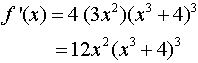Example 8: Find the derivative of function f given bySolution to Example 8:
Function f is of the form U3 where U = (x - 1) / (x + 3). Apply the chain rule to obtain f ' as follows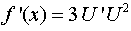Calculate U ', using the quotient rule, and substitute to obtainExpand and group like terms to obatin a final form for the derivative f 'Example 9: Find the derivative of function f given bySolution to Example 9:
The given function is of the form sqrt U with U = x3 + 2 x + 1. Calculate U ' and use the chain rule to obtainExample 10: Find the derivative of function f given bySolution to Example 10:
The given function is of the form U3/2 with U = x2 + 5. Apply the chain rule as followsCalculate U ', substitute and simplify to obtain the derivative f '.Example 11: Find the derivative of function f given bySolution to Example 11:
Function f is of the form U1/4 with U = (x + 6)/(x + 5). Use the chain rule to calculate f ' as follows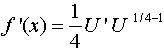Since U is the quotient of two function, use the quotient rule to find U ' and substitute to obtainExpand and group like termsChange the negative exponent into a positive exponent to find a final form of f ' as followsExercises: Find the derivative of each of the following functions.